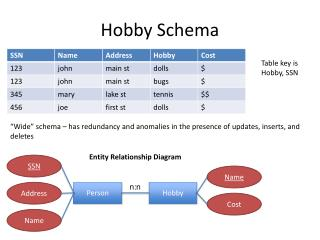DownloadDownload PresentationHobby Schema

# Hobby Schema

Télécharger la présentation## Hobby Schema

- - - - - - - - - - - - - - - - - - - - - - - - - - - E N D - - - - - - - - - - - - - - - - - - - - - - - - - - -
##### Presentation Transcript

1. Hobby Schema Table key is Hobby, SSN “Wide” schema – has redundancy and anomalies in the presence of updates, inserts, and deletes Entity Relationship Diagram SSN Name n:n Person Hobby Address Cost Name

2. Schema From ER Diagram SSNName, Address HobbyCost Schema is free of anomalies and redundancy Entity Relationship Diagram SSN Name n:n Address Person Hobby Hobby, SSN  Hobby, SSN Cost Name

3. Functional Dependencies • X  Y • Attributes X uniquely determine Y • I.e. for every pair of instances x1, x2 in X, with y1, y2 in Y, if x1=x2, y1=y2 • For Hobbies, we have: • SSN, Hobby  Name, Addr, Cost • SSN  Name, Addr • Hobby  Cost

4. Boyce-Codd Normal Form (BCNF) • For a relation R, with FDs of the form XY, every FD is either: 1) Trivial (e.g., Y contains X), or 2) X is a superkey; that is, it is a superset of the key of the table • If an FD violates 2), multiple rows with same X value may occur • Indicates redundancy, as rows with given X value all have same Y value • E.g., SSN  Name, Addr in non-decomposed hobbies schema • Name, Addr repeated for each appearance of a given SSN • To put a schema into BCNF, create subtables of form XY • E.g., tables where key is left side (X) of one or more FDs • Repeat until all tables in BCNF

5. BCNFify BCNFify(schema R, functional dependency set F): D = {(R,F)} // D is set of output relations while there is a (schema ,FD set) pair (S,F') in D not in BCNF, do: given XY as a BCNF-violating dependency in F’ replace (S,F’) in D with S1 = (XY,F1) and S2 = ((S-Y) U X, F2) where F1 and F2 are the FDs in F’ over XY or (S-Y) U X, respectively End return D

6. BCNFify Example for Hobbies Did we lose S,H  N,A,C? No! S,H  N, A, C is implied by HC and SN,A, since: 1) HC can be extended to: S,H  C, S 2) S  N, A can be extended to: S, H  N, A, H Since S,H is on left sides of both, can take union, giving S, H  C, S, N, A, H Armstrong’s Axioms Iter 1 S = SSN, H = Hobby, N = Name, A = Addr, C = Cost Iter 2 violates bcnf violates bcnf key S,H  N, A, C implies S,H  C 3 remaining tables are same as in ER decomposition